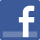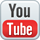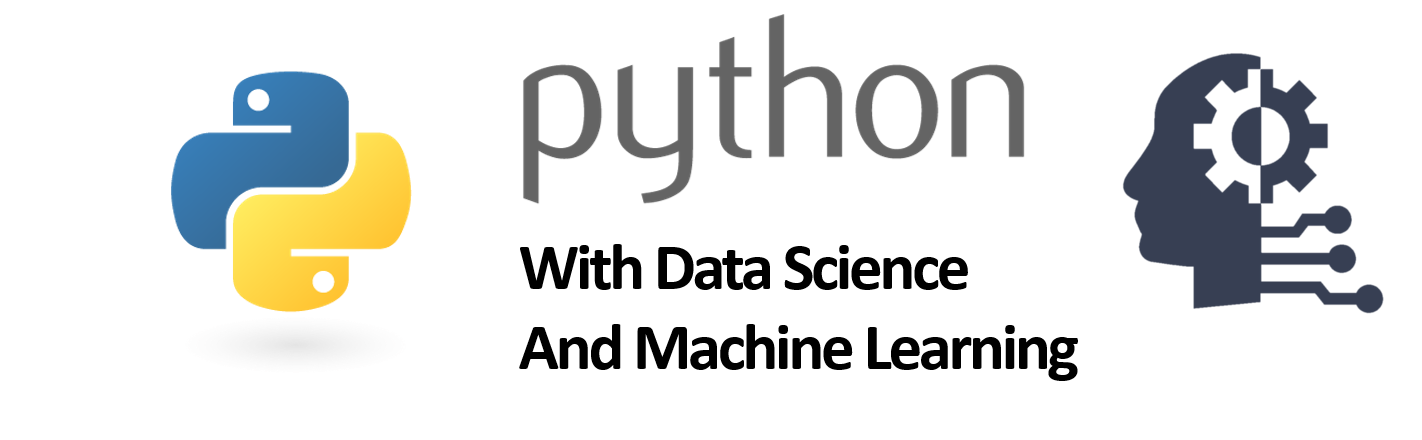﻿ Python with Data Science and Machine Learning computer training institue in West Delhi
• Facebook• Youtube##Professional Training & 100% Projects Based Training

Be Professionals From Professionals               Only Professionals can Make u Professional
• Part Time
• Duration           :      4 Months
• Class                :      1.30 Hrs Daily
• Days                 :      Mon-Fri.
• Half Time
• Duration           :      2 months
• Class                :      3.00 Hrs Daily
• Days                 :      Mon-Fri.
• Full Time
• Duration           :      1 month
• Class                :      4.30 Hrs Daily
• Days                 :      Mon-Fri.

## Course Description:

Python with Data Science and Machine Learning has been designed by industry experts that will be your guide to learning how to use the power of Python to analyze data, create beautiful visualizations, and use powerful machine learning algorithms. It covers a detailed overview of various algorithms and techniques, such as regression, classification, time series modeling, supervised and unsupervised learning, Natural Language Processing, etc. You will also learn how to program with Python, how to create amazing data visualizations, and how to use Machine Learning with Python. Not only that, we will mentor you on your Final Project work and provide useful insights to solve industry level problems in the real-business world. Here, you will learn how data science projects are run, and what are the real-business project challenges that you can face as a Data Scientist / Analyst. Imagine how you would feel when you have the skills to analyse complex business data and you could make sales predictions or recommend the next business opportunity for your organization.

### Introduction to CORE Python

1. History and Background
2. Comparison with other Programming Languages
3. Installation and Environment Setup
• Working in IDLE and CMD
• Working in VS-Code
• Working in Jupyter Notebook (Anaconda)
• Writing your First Python Program

### Core Programming Fundamentals

1. Displaying a Message
2. Datatypes and Variables
3. String Operations
• Concatenation
• Using title(), upper(), lower(), lstrip(), rstrip(), strip()
4. Arithmetic Operations
• Add, Subtract, Multiply, Divide, Modulus
• Using ** and //
• Using Math Functions [import math] : sqrt(), factorial(), pow(), pi
1. Conditional Statements with Relational and Logical Operators
• If… Else
• If… Elif… Else
• Nested if… Else
1. Loops and Ranges
• While loop
• range() and for loop
• Creating Patterns
• Using break, continue and pass statements
1. Basic Data Structures
1. List using [ ]
• Using append(), extend(), insert(), remove(), pop(), clear(), index(), count(), sort(), reverse(), copy(), len(), max(), min(), range()
1. Slicing a List using [_:_:_] notation
2. Tuples using ( )
1. Dictionary using { key : value }
• Using clear(), copy(), fromkeys(), get(), items(), keys(), popitem(), setdefault(), pop(), len(), del
1. Set using { }

### Comprehension

• List Comprehension
• Dictionary Comprehension
• Set Comprehension

### Working with Strings

• String representation and structure
• String input and output
• String Slicing
• String Functions
1. Using len(), str(), upper(), lower(), format(), find(), replace()
2. Using in and not in operators

### Functions

• Definition and Types of Function
• Working with Global and Local Variables
• Anonymous Function
1. Lambda
2. Filter, map, reduce
• Recursive Function
• Using *args and **kwargs
• Generators

### Modules and Packages

• Introduction to Modules
• Importing a module
• Working with math and os modules
• Working with datetime module
1. Using now(), today()
• Introduction to Packages
• Working with packages

Exception Handling

• Definition and Example of Exception
• Using try and except block
• Using raise keyword
• Using finally block
• Using try with else block

### File Handling

• Definition
• Opening and Closing a File
• Writing and Reading a File
• File Methods

### Object Oriented Programming

• Definition
• Classes and Objects
• Encapsulation
• Inheritance
• Polymorphism

### Regular expressions

• Introduction to CFG
• Match Function
• Search Function
• Matching VS Searching
• Patterns

### Introduction to Data Science

• What is analytics and data science?
• Overview of data science and analytics.
• Why analytics is becoming popular now?
• Application of analytics in business.
• Analytics vs data warehousing and MIS reporting.
• Various terminologies in analytics.
• How business are using the power of analytics?
• Various analytics tools and their usage.

### Introduction to Data Science with Python

• Installing python anaconda distribution.
• Python native data types.
• Basic programming concepts.
• Python data science packages overview.

### Python Basics: Basics Syntax, Data Structures

• Python objects
• Math and comparison operators
• Conditional statements
• Loops
• Functions
• Exception handling

### Python Concepts (Core)

• Overview and history of python.
• Python installation.
• Introduction to python editors & IDE’S (canaopy, pycharm, jupyter, rodeo etc.)
• Understand jupyter notebook & customize settings.
• Concept of packages / libraries – important packages (numpy, scipy, scikit-learn, pandas, matplotlib etc).
• Data types & data objects/ structure (strings, tuples, lists, dictionaries).
• List & dictionary comprehensions.
• Variables & value labels – date, times & values.
• Basic operations – mathematical – string – date.
• Simple plotting.
• Control flow & conditional statements.
• Debugging & code profiling.
• How to create class & modules and how to call them ?

### Numpy Package

• What is numpy?
• Importing numpy
• Numpy overview
• Numpy array creation & basic operation
• Numpy universal function.
•  Selecting & retrieving data.
• Data slicing
• Iterating numpy data.
• Shape manipulation.
• Stacking & splitting arrays.
• Copies and views : no copy, shallow copy, deep copy.
• Indexing : arrays of indices, Boolean arrays.

### Introduction to Pandas

• Selecting data from pandas data frame.
• Slicing & dicing using pandas.
• Group by/ aggregate.
• Strings with pandas.
• Cleaning up messy data with pandas.
• Dropping entries.
• Selecting entries

### Data Manipulation using Pandas

• Data alignment
• Sorting and ranking
• Summary statistics
• Missing values
• Merging data
• Concatenation
• Combining DataFrames.
• Pivot
• Duplicates
• Binning

### Pandas Package

• Importing pandas
• Pandas overview
• Object creation: series object, data frame object
• View data
• Selecting data by label & position
• Data slicing
• Boolean indexing
• Setting data

### Python Advance : Data Mugging with Pandas

• Applying functions data
• Histogramming
• String methods
• Merge data: concat, join & append
• Grouping & aggregation
• Reshaping.
• Analyzing data for missing values.
• Filling missing values : fill with constant, forward filling, mean.
• Removing duplicates
• Transforming data

### Python Advance: Visualization with Matplotlib

• Anatomy of a matplotlib plot.
• Matplotlib basics plots and it’s containers.
• A matplotlib figure, it’s components and properties.
• Axes and other graphical objects.
• Pylab and pyplot.
• Data and matplotlib plots.
• What is subplot?
• Modifying size of figures.
• Plotting size of figures.
• Plotting routines with pyplot.
• Deleting an axes.
• Setting up plot title, axes labels, legend, layout.
• Showing, saving and closing your plot.
• Save a plot to an image file and pdf file.
• Use Cla (). Clf () or close

### Introduction to statistics

• Two areas of statistics in data science.
• Descriptive statistics.
• Inferential statistics.
• Statistics terms and definitions.
• Type of data
• Quantitative vs qualitative data
• Data measurement scales.

### Harnessing Data

• Sampling data, with and without replacement.
• Sampling  methods, random vs non – random
• Measurement on samples.
• Random sampling methods.
• Simple random, stratified, cluster, systematic sampling.
• Biased vs unbiased sampling.
• Sampling error
• Data collection methods.

### Exploratory Analysis

• Measures of central tendencies.
• Mean, median and mode
• Data variability : range, quartiles, standard deviation
• Calculating standard deviation.
• Z-score/ standard score
• Empirical rule
• Calculating percentiles.
• Outliners

### Distributions

• Distribution introduction
• Normal distribution
• Central limit theorem
• Histogram – normalization
• Other distribution : poisson, binominal etc.
• Normality testing
• Skewness
• Kurtosis
• Measure of distance
• Euclidean, manhattan and minkowski distance.

### Hypothesis and computational techniques

• Hypothesis testing
• Null hypothesis, p- value
• Need for hypothesis testing in business.
• Two tailed, left tailed and right tailed test.
• Hypothesis testing outcomes : type 1 &2 errors.
• Parametric test, t – tests : one sample, two sample, paired.
• One way ANOVA.
• Importance of parametric tests
• Non parametric tests : chi- square, mann – whitney, Kruskal- wallis etc.
• Which test to choose?
• Ascertaining accuracy of data

### Correlation and Regression

• Introduction to regression
• Types of regression
• Hands on of a regression with python
• Correlation
• Weak and strong correlation
• Finding correlation with python .

### Introduction to Machine Learning

• What is machine learning?
• Data science vs machine learning.
• Fundamentals of machine learning.
• Converting business problems to data problems.
• Understand supervised and unsupervised learning with examples.
• Understand biases associated with my machine learning algorithm.
• Drivers of machine learning algorithms.
• Cost functions.
• Brief introduction to gradient descent.
• Importance of model validation.
• Model performance metrics.

### Unsupervised Learning

• K-means clustering : theory, Euclidean method
• K-means hands on with python.

### Supervised Learning

• Simple linear regression : implementing in python, working on use case.
• Multiple linear regression : implementing in python, working on use case.
• K- nearest neighbor: implementing in python, KNN advantages, working on use case.
• Decision trees: implanting in python, decision trees pros and cons, working on use case.

• Tuning with hyper parameters.
• Popular machine learning algorithms.
• Clustering, classification and regression.
• Supervised vs unsupervised.
• Choice of machine learning algorithms.
• Grid search vs random search cross validation.

### Principle Component Analysis (PCA)

• Key concepts of dimensionality reduction.
• PCA theory
• Hands on coding
• Case study on PCA

### Random Forest – Ensemble

• Key concepts of random forest.
• Hands on coding
• Pros and cons
• Case study on random forest

### Support Vector Machine (SVN)

• Key concepts of support vector machine.
• Hands on coding.
• Pros and cons,
• Text processing with vectorization
• Sentiment analysis with textBlob.

### Naïve Bayes Classifier

• Key concept of Naïve Bayes.
• Hands on coding
• Pros and cons
• Naïve Bayes for test classification.
• New articles tagging.

### Recommender System

• User-Based Collaborative Filtering
• Itembased collaborative filtering
• Finding moviesimilarities
• Improving the results
• Making movie recommendations
• Improving the results

### Apache SPARK - Machine Learning on Big Data

• Installing SPARK
• Introduction and Overview
• Resilient Distributed Dataset
• MLLib
• SPARK Decision trees
• K-Means Clustering
• TF / IDF
• Searching Wikipedia with SPARK

Python Training center in West Delhi, Uttam nagar. Best certified computer course curriculum for professional learning institute near Dwarka, Uttam Nagar, Jankapuri, Tilak Nagar, Subhash Nagar, Tagore Garden, Rajouri Garden, Ramesh Nagar, Moti Nagar, Kirti Nagar, Shadipur, Patel Nagar, Rajendra Place, Karol Bagh, Jhandewalan, Ramakrishna Ashram, Connaught Place, vikaspuri, najafgarh, Delhi Cantt, Dhaula Kuan, Palam Colony, Palam Villag, Dabri, Hari Nagar, Fateh Nagar, Raja Garden, Punjabi Bagh, Shivaji Park, Paschim Vihar, Peeragarhi, Inderlok, Netaji Subhash Place, Pitampura, Rohini, Chandni Chowk, New Delhi, East Delhi, North Delhi, West Delhi.

﻿

### Message Us

Max Chars Allowed = 100